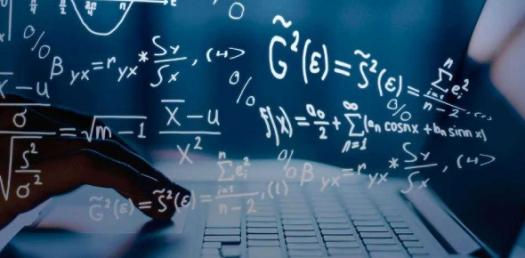# Let's Test Your General Math Skill With Quiz

25 Questions | Attempts: 1705
ShareSettingsSome people get a hard time when it comes to math and this is mostly due to one not having adequate practice. When you give your all to your studies, one thing you get are good results. Do you wish to test your general math skills with the quiz below? Let’s do it and share your score in the comment section.

• 1.
Which is the solution to the equation 5(x + 4) = 60
• A.

16

• B.

-16

• C.

8

• D.

-8

• 2.
Which inequality states "x is less than 10"?
• A.

10 > x

• B.

X > 10

• 3.
Which fraction is equivalent to 3x  ?  8
• A.

9x/ 32

• B.

1x/72

• C.

8/3x

• D.

6x/16

• 4.
Describe the location of the point (-3, 5)
• A.

• B.

• C.

• D.

• 5.
Solve for x.  2 x = 20 3
• A.

30

• B.

-30

• C.

13.3

• 6.
Choose an ordered pair that is a solution set for the equation 2x + 20 = 4y + 4
• A.

(5, 2)

• B.

(2, 5)

• C.

(-2, -5)

• D.

(-5, 2)

• 7.
Which expression has a value of 121?
• A.

20 *(5 + 7) * 3

• B.

20 + 5 * 4 + 21

• C.

20 * 5 + 7 * 3

• 8.
3(x + 6) = 6x + 21
• A.

-1

• B.

1

• C.

13

• D.

-13

• 9.
Solve the proportion6   =    821       z
• A.

-28

• B.

28

• C.

12

• D.

24

• 10.
What is the decimal equivalent to 8/12? (Round to the hundredths place)
• A.

1.50

• B.

.66

• C.

0.67

• 11.
An iPhone costs \$300.  The sales tax is 9%.  What is the total cost of the iPhone?
• A.

\$309

• B.

\$27

• C.

\$336

• D.

\$327

• 12.
11.4 is 9.5% of what number?
• A.

1.0

• B.

12

• C.

100

• D.

120

• 13.
What is the slope of a line through the points (-4, -8) (0, 2)
• A.

-5/2

• B.

2/5

• C.

5/2

• D.

-2/5

• 14.
What is the y- intercept of the graph that crosses through the points (0, 1) (10, -2)
• A.

1

• B.

-2

• C.

10

• D.

-1

• 15.
A map has a scale of 2 cm : 26 mi.  What is the actual distance if the distance on the map is 5 cm?
• A.

65 cm

• B.

65 mi

• C.

.38 cm

• D.

.38 mi

• 16.
What is the slope of a line that is parallel to the equation 4x + 5y = 15
• A.

4/5

• B.

-4/5

• C.

5

• D.

-5

• 17.
What is the slope of the line that runs perpendicular to the equation y = 3/10x + 7 and crosses through the point (0, 5)?
• A.

10/3

• B.

7

• C.

-10/3

• D.

1/7

• 18.
Simplify the expression: 5c – 6 – (7c + 8)
• A.

-12c + 2

• B.

2c + 2

• C.

2c - 14

• D.

-2c - 14

• 19.
• A.

8 1/5

• B.

8 13/24 lb

• C.

8 -1/5

• 20.
Find the base of the trapezoid with the following dimensions: h = 6base 1 = 26 base 2 = ? area = 126Round your answer to the nearest integer.
• A.

15

• B.

16

• C.

6

• D.

5

• 21.
What is the radius of a circle with an area of 1661 sq inches?
• A.

529

• B.

528

• C.

23

• D.

24

• 22.
Which statement describes the inequality x > - 3
• A.

X can be any number less than or equal to - 3

• B.

X can be any number greater than or equal to 3

• C.

X can be any number greater than - 3

• D.

X can be any number greater than or equal to - 3

• 23.
The volume of a cube is 125 square cm.  What is the length, width and height?
• A.

5 cm

• B.

41.2 cm

• C.

20.8 cm

• D.

10 cm

• 24.
You ate 14/20  of a pizza.  What remains?
• A.

6/20

• B.

1/20

• C.

3/10

• D.

3/4

• 25.
Your 4- digit password contains 2 digits and 2 lowercase letters.  If you can repeat the numbers and letters, how many possible combinations exist for the password?
• A.

58,500

• B.

67, 600

• C.

16

• D.

10,000

## Related TopicsBack to top
×

Wait!
Here's an interesting quiz for you.